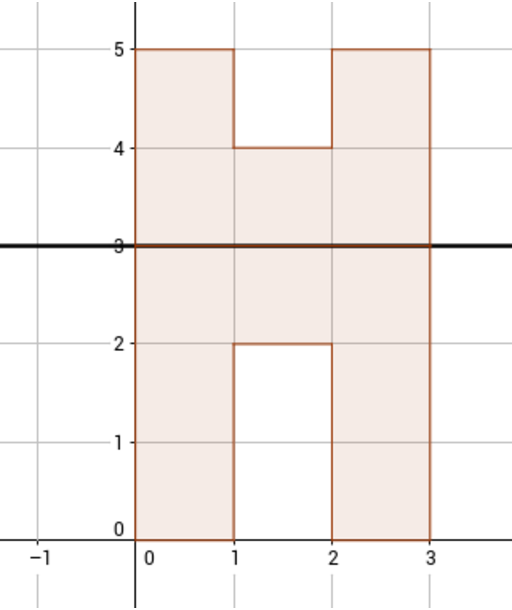시간 제한메모리 제한제출정답맞힌 사람정답 비율
2 초 512 MB111100.000%

## 문제

Petya was getting bored at his Math lesson, so he started painting squares at his grid paper. When he got bored of that action as well, he noticed that he now has a connected set of k painted squares — it is possible to get from any painted square to any other painted one by moving between painted squares sharing a common side.

He cut the figure away from the sheet of paper and folded it along some grid line (horizontal or vertical, he didn't remember). After that he painted squares of some other grid paper to create the copy of the folded figure. Now Petya has lost his original figure, so all he has is the copy of it after folding. Now Petya wants to restore the figure.

It is difficult to restore the figure exactly, but Petya has decided that he would like to have at least some figure of k squares that can be folded to get the same folded image. Help him, find some connected figure of k squares that can be folded the required way.

Consider for example the second sample test. It has a folded figure that is similar to an upside-down "U" letter, the original figure contains 12 squares. One way the original figure could look like is shown at the picture, it was folded along a line y = 3:## 입력

Input data contains multiple test cases. The first line contains the number of test cases t (1 ≤ t ≤ 200).

Each of the t test cases is described in the following way: the first line of the description contains two integers n, k — the number of painted squares in the folded figure and the number of painted squares in the original figure (1 ≤ n < k ≤ 105).

Each of the following n lines contains two integers xi, yi — coordinates of the i-th painted square's bottom left corner (-108 ≤ xi, yi ≤ 108). It is guaranteed that all painted cells are distinct and form a connected figure.

It is guaranteed that the sum of values of k in all test cases of one input data doesn't exceed 105.

## 출력

For each test case output the answer to this test. Output the description of the figure and the way to fold it to get the input figure.

The first line must contain the description of the folding line. The following k lines must contain two integers (x'i, y'i) each — the coordinates of the squares of the connected figure that can be folded along the given line to get the figure from the input data.

The folding line description must be one of the following 4:

• L num — fold along the line x = num, put left side atop the right one;
• R num — fold along the line x = num, put the right side atop the left one;
• U num — fold along the line y = num, put the top side atop the bottom one;
• D num — fold along the line y = num, put the bottom side atop the top on.

All x'i, y'i, and the coordinate of the folding line must not exceed 109 by their absolute values. It is guaranteed that the required figure exists. If there are several possible answers, output any of them.

## 예제 입력 1

2
7 14
0 0
0 1
0 2
1 2
2 2
2 1
2 0
7 12
0 0
0 1
0 2
1 2
2 2
2 1
2 0


## 예제 출력 1

L 0
0 0
0 1
0 2
1 2
2 0
2 1
2 2
-1 0
-1 1
-1 2
-2 2
-3 2
-3 1
-3 0
U 3
0 0
0 1
0 2
1 2
2 2
2 1
2 0
0 3
1 3
2 3
0 4
2 4